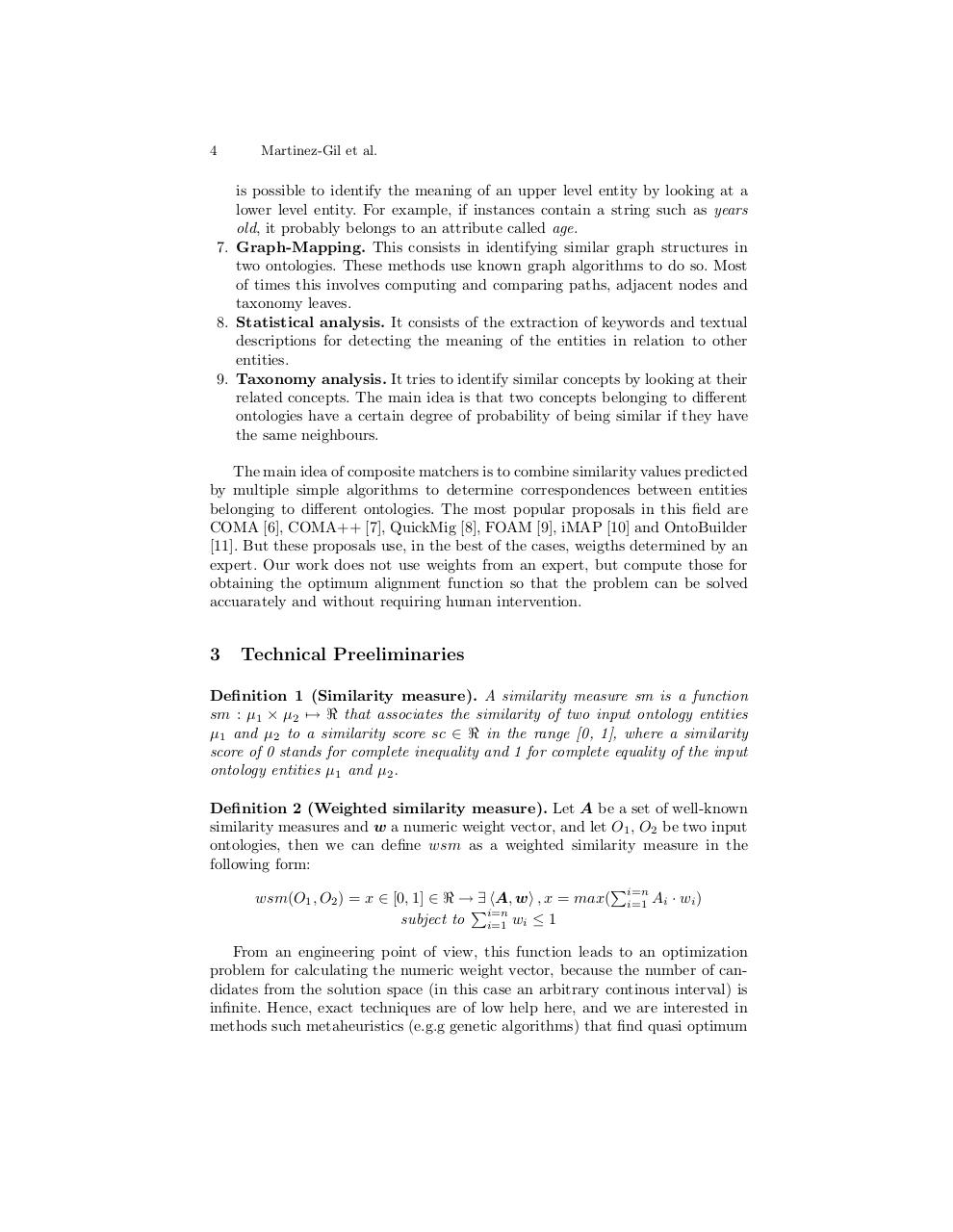# Ontology Matching Genetic Algorithms.pdfPage 1 2 3 45615

#### Text preview

4

Martinez-Gil et al.

is possible to identify the meaning of an upper level entity by looking at a
lower level entity. For example, if instances contain a string such as years
old, it probably belongs to an attribute called age.
7. Graph-Mapping. This consists in identifying similar graph structures in
two ontologies. These methods use known graph algorithms to do so. Most
of times this involves computing and comparing paths, adjacent nodes and
taxonomy leaves.
8. Statistical analysis. It consists of the extraction of keywords and textual
descriptions for detecting the meaning of the entities in relation to other
entities.
9. Taxonomy analysis. It tries to identify similar concepts by looking at their
related concepts. The main idea is that two concepts belonging to different
ontologies have a certain degree of probability of being similar if they have
the same neighbours.
The main idea of composite matchers is to combine similarity values predicted
by multiple simple algorithms to determine correspondences between entities
belonging to different ontologies. The most popular proposals in this field are
COMA , COMA++ , QuickMig , FOAM , iMAP  and OntoBuilder
. But these proposals use, in the best of the cases, weigths determined by an
expert. Our work does not use weights from an expert, but compute those for
obtaining the optimum alignment function so that the problem can be solved
accuarately and without requiring human intervention.

3

Technical Preeliminaries

Definition 1 (Similarity measure). A similarity measure sm is a function
sm : µ1 × µ2 7→ &lt; that associates the similarity of two input ontology entities
µ1 and µ2 to a similarity score sc ∈ &lt; in the range [0, 1], where a similarity
score of 0 stands for complete inequality and 1 for complete equality of the input
ontology entities µ1 and µ2 .
Definition 2 (Weighted similarity measure). Let A be a set of well-known
similarity measures and w a numeric weight vector, and let O1 , O2 be two input
ontologies, then we can define wsm as a weighted similarity measure in the
following form:
Pi=n
wsm(O1 , O2 ) = x ∈ [0, 1] ∈ &lt; → ∃ hA, wi , x = max( i=1 Ai · wi )
Pi=n
subject to i=1 wi ≤ 1
From an engineering point of view, this function leads to an optimization
problem for calculating the numeric weight vector, because the number of candidates from the solution space (in this case an arbitrary continous interval) is
infinite. Hence, exact techniques are of low help here, and we are interested in
methods such metaheuristics (e.g.g genetic algorithms) that find quasi optimum